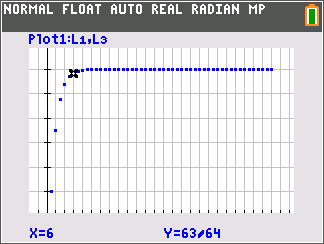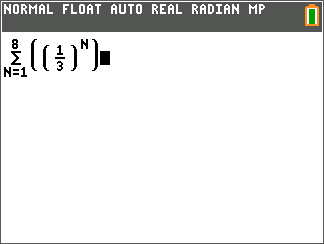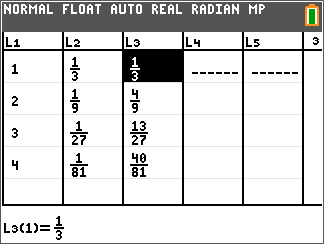# Activities

••• ##### Subject Area

• Math: Calculus: Series and Taylor Polynomials

• ##### Author9-12

45 Minutes

• ##### Device
• TI-84 Plus
• TI-84 Plus Silver Edition
•TI-84 Plus C Silver Edition
•TI-84 Plus CE

## Summing Up Geometric Series#### Activity Overview

In this activity, students will explore infinite geometric series and the partial sums of geometric series. The students will determine the limits of these sequences and series using tables and graphs.

#### Key Steps

•Students will find a partial sum of two geometric series.

•Students will graph the series by generating a list with the cumulative sums of the terms of the sequence.

•Students will find the sum of a geometric series by taking the limit of the nth sum.